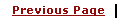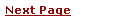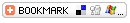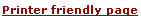C - Bits ManipulationsLearning C
C Function References
C Useful Resources# C - Bits ManipulationBit manipulation is the act of algorithmically manipulating bits or other pieces of data shorter than a byte. C language is very efficient in manipulating bits.

Here are following operators to perform bits manipulation:

## Bitwise Operators:

Bitwise operator works on bits and perform bit by bit operation.

Assume if B = 60; and B = 13; Now in binary format they will be as follows:

A = 0011 1100

B = 0000 1101

-----------------

A&B = 0000 1000

A|B = 0011 1101

A^B = 0011 0001

~A  = 1100 0011

Show Examples

There are following Bitwise operators supported by C language

OperatorDescriptionExample
& Binary AND Operator copies a bit to the result if it exists in both operands. (A & B) will give 12 which is 0000 1100
| Binary OR Operator copies a bit if it exists in eather operand. (A | B) will give 61 which is 0011 1101
^ Binary XOR Operator copies the bit if it is set in one operand but not both. (A ^ B) will give 49 which is 0011 0001
~ Binary Ones Complement Operator is unary and has the efect of 'flipping' bits. (~A ) will give -60 which is 1100 0011
<< Binary Left Shift Operator. The left operands value is moved left by the number of bits specified by the right operand. A << 2 will give 240 which is 1111 0000
>> Binary Right Shift Operator. The left operands value is moved right by the number of bits specified by the right operand. A >> 2 will give 15 which is 0000 1111

The shift operators perform appropriate shift by operator on the right to the operator on the left. The right operator must be positive. The vacated bits are filled with zero.

For example: x << 2 shifts the bits in x by 2 places to the left.

 ```if x = 00000010 (binary) or 2 (decimal) then: x >>= 2 => x = 00000000 or just 0 (decimal) Also: if x = 00000010 (binary) or 2 (decimal) then x <<= 2 => x = 00001000 or 8 (decimal) ```

Therefore a shift left is equivalent to a multiplication by 2. Similarly a shift right is equal to division by 2. Shifting is much faster than actual multiplication (*) or division (/) by 2. So if you want fast multiplications or division by 2 use shifts.

To illustrate many points of bitwise operators let us write a function, Bitcount, that counts bits set to 1 in an 8 bit number (unsigned char) passed as an argument to the function.

 ```int bitcount(unsigned char x) { int count; for ( count=0; x != 0; x>>=1); { if ( x & 01) count++; } return count; } ```

This function illustrates many C program points:

• for loop not used for simple counting operation.

• x >>= 1 => x = x>> 1;

• for loop will repeatedly shift right x until x becomes 0

• use expression evaluation of x & 01 to control if

• x & 01 masks of 1st bit of x if this is 1 then count++

# Bit Fields

Bit Fields allow the packing of data in a structure. This is especially useful when memory or data storage is at a premium. Typical examples:

• Packing several objects into a machine word. e.g. 1 bit flags can be compacted.

• Reading external file formats -- non-standard file formats could be read in. E.g. 9 bit integers.

C allows us do this in a structure definition by putting :bit length after the variable.For example:

 ```struct packed_struct { unsigned int f1:1; unsigned int f2:1; unsigned int f3:1; unsigned int f4:1; unsigned int type:4; unsigned int my_int:9; } pack; ```

Here the packed_struct contains 6 members: Four 1 bit flags f1..f3, a 4 bit type and a 9 bit my_int.

C automatically packs the above bit fields as compactly as possible, provided that the maximum length of the field is less than or equal to the integer word length of the computer. If this is not the case then some compilers may allow memory overlap for the fields whilst other would store the next field in the next word.